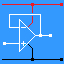RANDOM PAGE

SITE SEARCH

LOG
IN

HELP

# Calculator Friendly Formulas

This is the AQA version closing after June 2019. Visit the the version for Eduqas instead.

This page shows how formulas match calculator key presses. On different models of calculator you will findwhich is the same as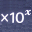.

### Calculator Key Presses

Ohm's Law
R = V / I        12 V / 10mA VI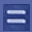12103V = I R        22mA x 3.3kΩ IR2233.33I = V / R        12 / 22kΩ VR12223Power Calculations
P = I V        10mA x 7V IV1037P = I2 R        10mA2 x 100Ω IR102100P = V2 / R        122 / 8 VR128P = VRMS2 / R        (12 x 0.7)2 / 8 VR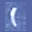120.7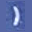8Parallel Resistors / Series Capacitors
1 / Rt = 1/R1 + 1/R2 11R11R2Voltage Divider
Vout = Vs R2 / (R1 + R2) VsR2R1R2Amplifier Gain
Gv = Vout / Vin VoutVinAstable Frequency
F = 1.44 / ((Ra + 2Rb) C1) 1.44Ra2RbC1F = 1.44 / ((10K+ 2 x 100K) x 5nF) 1.441032100359reviseOmatic V3     Contacts, ©, Cookies, Data Protection and Disclaimers Hosted at linode.com, London